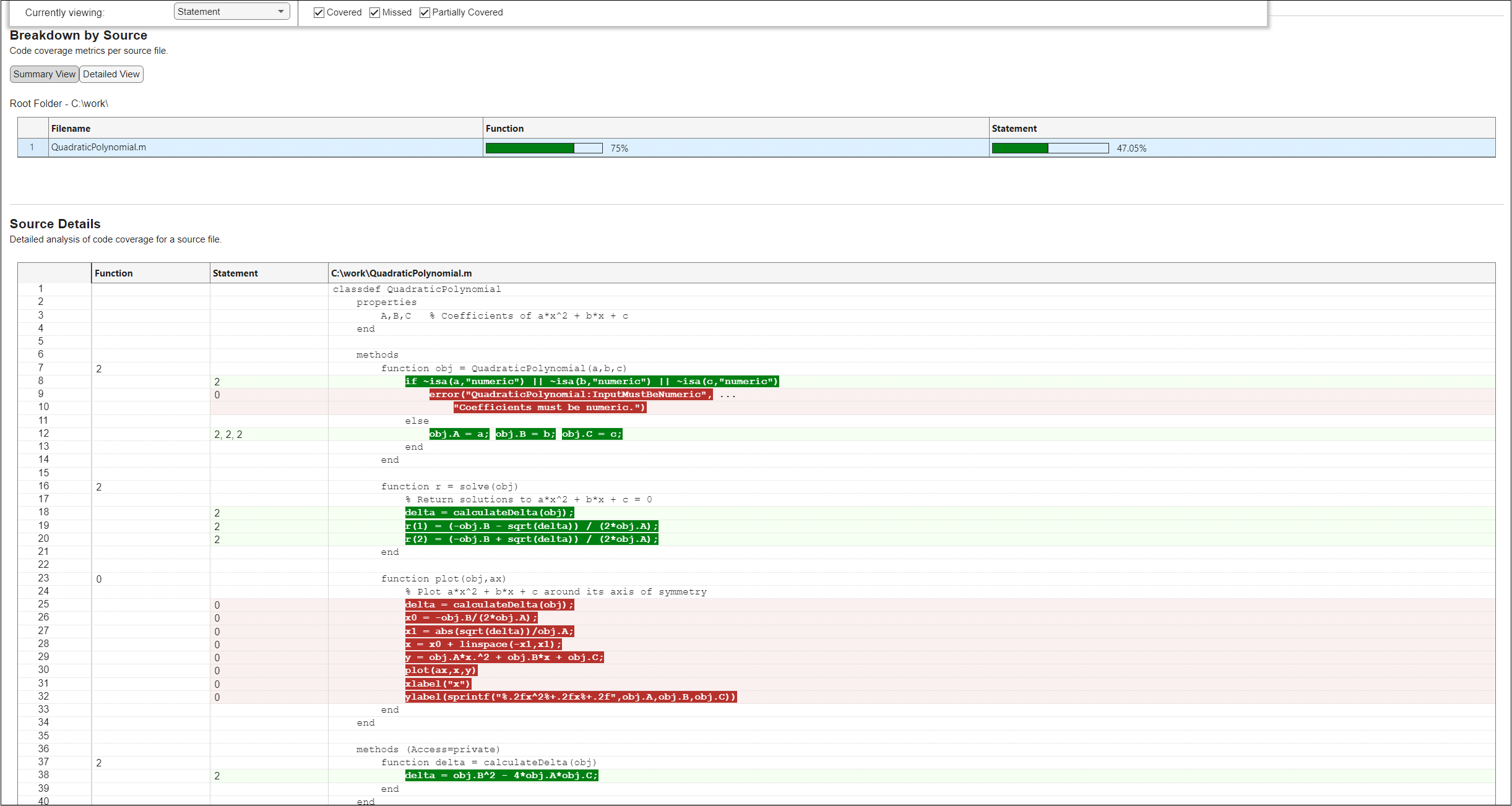# Collect Statement and Function Coverage Metrics for MATLAB Source Code

When you run tests, you can collect and access code coverage information for your MATLAB® source code by adding an instance of the `matlab.unittest.plugins.CodeCoveragePlugin` class to the test runner. In MATLAB, a plugin created using a `matlab.unittest.plugins.codecoverage.CoverageResult` or `matlab.unittest.plugins.codecoverage.CoverageReport` format object provides information about statement and function coverage. For more information about statement and function coverage, see Types of Code Coverage for MATLAB Source Code.

This example shows how to collect, programmatically access, and generate reports including statement and function coverage information for source code in a file. The file defines the `QuadraticPolynomial` class, which represents quadratic polynomials. The class constructor first validates that the coefficients of the polynomial are numeric values and then uses these values to initialize the class properties. The class includes the `solve` method to return the roots of the specified quadratic polynomial, and the `plot` method to plot the polynomial around its axis of symmetry. To view the complete code for `QuadraticPolynomial`, see `QuadraticPolynomial` Class Definition.

### Run Tests and Analyze Code Coverage Information

In your current folder, save the `QuadraticPolynomial` class definition in a file named `QuadraticPolynomial.m`. Then, create the `QuadraticPolynomialTest1` test class in your current folder. Add two `Test` methods to the class that test the `solve` method against real and imaginary solutions.

```classdef QuadraticPolynomialTest1 < matlab.unittest.TestCase methods (Test) function realSolution(testCase) p = QuadraticPolynomial(1,-3,2); actSolution = p.solve(); expSolution = [1 2]; testCase.verifyEqual(actSolution,expSolution) end function imaginarySolution(testCase) p = QuadraticPolynomial(1,2,10); actSolution = p.solve(); expSolution = [-1-3i -1+3i]; testCase.verifyEqual(actSolution,expSolution) end end end ```

To run tests and perform a code coverage analysis, first create a test runner with a plugin that provides programmatic access to the statement and function coverage information for source code in the file `QuadraticPolynomial.m`.

```import matlab.unittest.plugins.CodeCoveragePlugin import matlab.unittest.plugins.codecoverage.CoverageResult runner = testrunner("textoutput"); format = CoverageResult; p = CodeCoveragePlugin.forFile("QuadraticPolynomial.m",Producing=format); addPlugin(runner,p)```

Create a test suite from the `QuadraticPolynomialTest1` class and run the tests. The tests pass.

```suite1 = testsuite("QuadraticPolynomialTest1"); run(runner,suite1);```
```Running QuadraticPolynomialTest1 .. Done QuadraticPolynomialTest1 __________ ```

After the test run, the `Result` property of the `CoverageResult` object holds the coverage result. Access the statement coverage summary from the coverage result. The returned vector indicates that the tests executed 8 out of the 17 statements in the file, resulting in 47% statement coverage. The statement coverage is low because the tests did not execute the code that throws an error and the code within the `plot` method.

```result1 = format.Result; statementSummary = coverageSummary(result1,"statement")```
```statementSummary = 1×2 8 17 ```

Access the function coverage summary. The summary indicates that the function coverage is 75% because the tests missed one of the four methods in the `QuadraticPolynomial` class (`plot`).

`functionSummary = coverageSummary(result1,"function")`
```functionSummary = 1×2 3 4 ```

Now, generate an HTML code coverage report from the coverage result. The report displays information about statement and function coverage and uses different colors to highlight the executed or missed statements and functions.

`generateHTMLReport(result1)`

You can interact with the HTML code coverage report. For example, in the Overall Coverage Summary section, you can select a coverage type from the Currently viewing list to view detailed information about that coverage type. This figure shows the Source Details section for statement coverage, which displays all the statements that were covered or missed.### Run Additional Tests to Improve Coverage

Create tests to execute the parts of the source code that were not executed by `suite1`. In your current folder, create another test class named `QuadraticPolynomialTest2`. Add a `Test` method to the class that tests against nonnumeric inputs and another `Test` method that tests properties of a plotted polynomial.

```classdef QuadraticPolynomialTest2 < matlab.unittest.TestCase methods (Test) function nonnumericInput(testCase) testCase.verifyError(@()QuadraticPolynomial(1,"-3",2), ... "QuadraticPolynomial:InputMustBeNumeric") end function plotPolynomial(testCase) p = QuadraticPolynomial(1,-3,2); fig = figure; testCase.addTeardown(@close,fig) ax = axes(fig); p.plot(ax) actYLabelText = ax.YLabel.String; expYLabelText = '1.00x^2-3.00x+2.00'; testCase.verifyEqual(actYLabelText,expYLabelText) end end end ```

Create a test suite from the `QuadraticPolynomialTest2` class and run the tests. The tests pass.

```suite2 = testsuite("QuadraticPolynomialTest2"); run(runner,suite2);```
```Running QuadraticPolynomialTest2 . ```
```. Done QuadraticPolynomialTest2 __________ ```

In this example, you ran different test suites to qualify the same source code. To report on the total aggregated coverage for these test runs, generate an HTML report from the union of the coverage results corresponding to the test runs. The report shows that the two test suites combined fully exercised the statements and functions in the source code.

```result2 = format.Result; generateHTMLReport(result1 + result2)```

### `QuadraticPolynomial` Class Definition

This code provides the complete contents of the `QuadraticPolynomial` class.

```classdef QuadraticPolynomial properties A,B,C % Coefficients of a*x^2 + b*x + c end methods function obj = QuadraticPolynomial(a,b,c) if ~isa(a,"numeric") || ~isa(b,"numeric") || ~isa(c,"numeric") error("QuadraticPolynomial:InputMustBeNumeric", ... "Coefficients must be numeric.") else obj.A = a; obj.B = b; obj.C = c; end end function roots = solve(obj) % Return solutions to a*x^2 + b*x + c = 0 delta = calculateDelta(obj); roots(1) = (-obj.B - sqrt(delta)) / (2*obj.A); roots(2) = (-obj.B + sqrt(delta)) / (2*obj.A); end function plot(obj,ax) % Plot a*x^2 + b*x + c around its axis of symmetry delta = calculateDelta(obj); x0 = -obj.B/(2*obj.A); x1 = abs(sqrt(delta))/obj.A; x = x0 + linspace(-x1,x1); y = obj.A*x.^2 + obj.B*x + obj.C; plot(ax,x,y) xlabel("x") ylabel(sprintf("%.2fx^2%+.2fx%+.2f",obj.A,obj.B,obj.C)) end end methods (Access=private) function delta = calculateDelta(obj) delta = obj.B^2 - 4*obj.A*obj.C; end end end ```